# Dispersion Problem and Its Solution - Dispersion-Compensating Fibers

Optical amplifiers solve the fiber-loss problem but, at the same time, make the dispersion problem worse because dispersive effects keep accumulating along the entire chain of amplifiers. Indeed, long-haul WDM systems making use of amplifiers are often limited by the dispersive and nonlinear effects rather than fiber losses. However, the dispersion problem can be managed in practice through a suitable dispersion-compensation scheme. The following series of tutorials focus on several such techniques.

All long-haul lightwave systems employ single-mode optical fibers in combination with distributed feedback (DFB) semiconductor lasers with a relatively narrow linewidth (<0.1 GHz). As discussed in the dispersion-induced pulse broadening tutorial, the performance of such systems is often limited by pulse broadening induced by group-velocity dispersion (GVD) of silica fibers. Direct modulation of a DFB laser chirps optical pulses in an optical bit stream and broadens their spectrum enough that direct modulation cannot be used at bit rates above 2.5 Gb/s.

WDM systems operating at channel bit rates of 10 Gb/s or more often employ external modulators to avoid spectral broadening induced by frequency chirping. Under such conditions, the GVD-limited transmission distance L at a given bit rate B is obtained from this equation:and is given by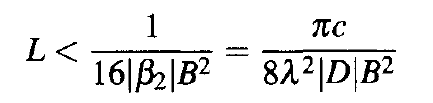where β2 is related to the commonly used dispersion parameter D through the following equation:For "standard" telecommunication fibers D is about 16 ps/(km-nm) near λ = 1.55 μm. The L equation above predicts that L cannot exceed 30 km at a bit rate of 10 Gb/s when such fibers are used for designing lightwave systems.

The existing worldwide fiber-cable network, installed during the 1980s, consists of more than 50 million kilometers of standard fiber. This fiber was suitable for second- and third-generation systems designed to operate at  bit rates of up to 2.5 Gb/s with repeater spacing of under 80 km (without optical amplifiers). However, the same fiber could not be used for upgrading the existing transmission links to fourth-generation systems (operating at 10 Gb/s and employing optical amplifiers for loss compensation) because of the 30-km dispersion limit set by the L equation above. Although it is possible to manufacture dispersion-shifted fibers, installation of new fibers is a costly solution to the dispersion problem, and it is not a viable alternative in practice. For this reason, several dispersion-management schemes were developed during the 1990s to address the upgrade problem.

One may think that the dispersion problem can be solved for new fiber links by employing dispersion-shifted fibers and operating the link close to the zero-dispersion wavelength of this fiber so that |D| ≈ 0. Under such conditions, system performance is limited by third-order dispersion (TOD). The dashed line in the following figure shows the maximum possible transmission distance at a given bit rate B when D = 0.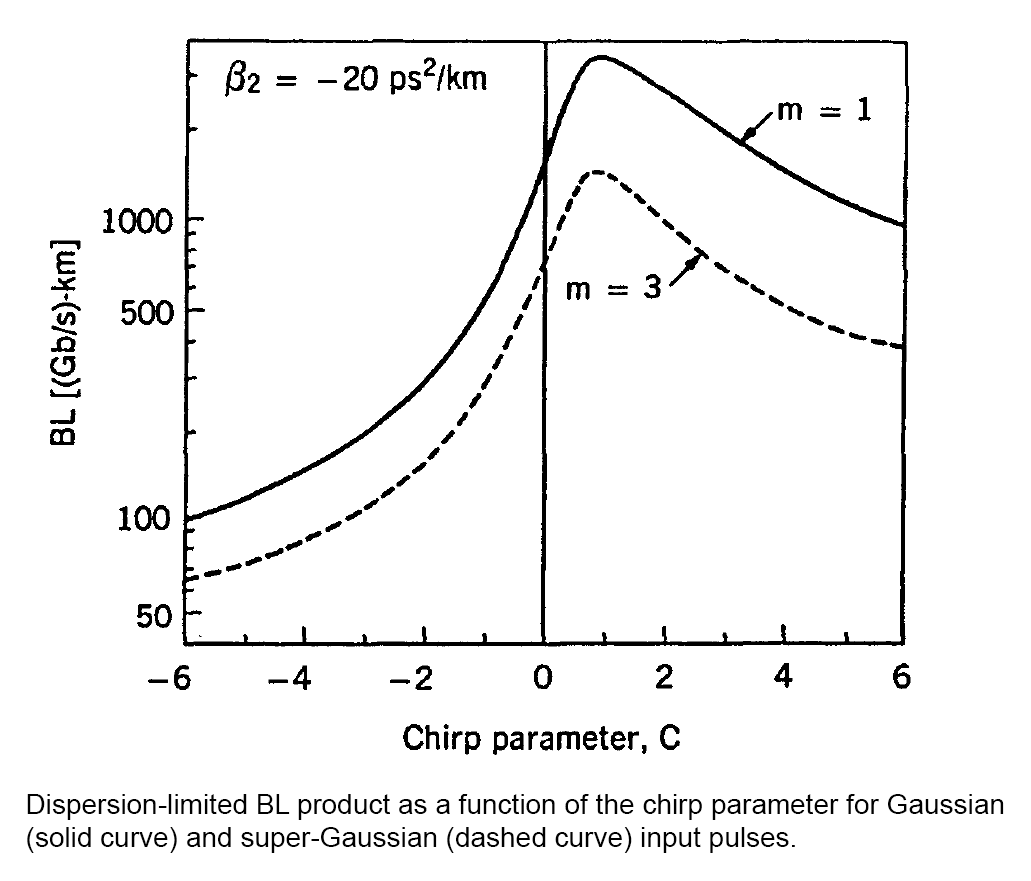Indeed, such a system can operate over more than 1,000 km even at a bit rate of 40 Gb/s. However, this solution is not practical for WDM systems because of four-wave mixing (FWM). As discussed in the nonlinear optical effects tutorial, the nonlinear phenomenon of FWM becomes quite efficient for low values of the dispersion parameter D and limits performance of any system operating close to the zero-dispersion wavelength of the fiber. For this reason, some form of dispersion management is employed for all long-haul WDM systems.

The basic idea behind any dispersion-management scheme is quite simple and can be understood from the pulse-propagation equation used in the dispersion-induced pulse broadening tutorial for studying the impact of fiber dispersion and written here as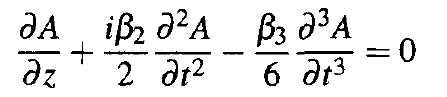where β3 governs the effects of third-order dispersion (TOD). This equation ignores the nonlinear effects that will be included later, assuming that signal power is low enough that all nonlinear effects can be neglected. It can be easily solved with the Fourier-transform method and has the solutionwhereis the Fourier transform of A(0, t).

Dispersion-induced degradation of the optical signal is caused by the z-dependent phase factor acquired by spectral components of the pulse during propagation inside the fiber. Indeed, one can think of fiber as an optical filter with the transfer function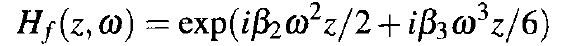All optical-domain dispersion-management schemes implement an optical "filter" whose transfer function H(ω) is chosen such that it cancels the phase factor associated with the fiber. As seen from A(z, t) expression above, if H(ω) = Hf*(L, ω), the output signal can be restored to its input form at the end of a fiber link of length L. Moreover, if nonlinear effects are negligible, such a filter can be placed at the transmitter end, or at the receiver end, or anywhere along the fiber link.

Consider the simplest situation shown schematically in the figure below, where a dispersion-compensating filter is placed just before the receiver.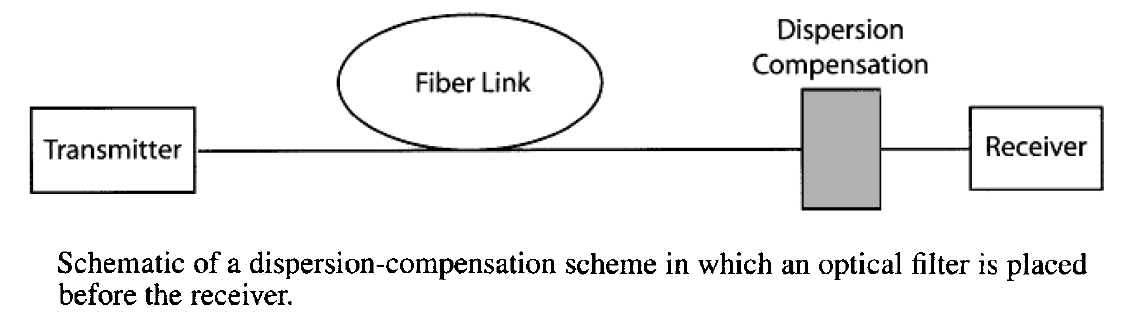From the A(z, t) expression above, the optical field after the filter is given byExpanding the phase of H(ω) in a Taylor series and retaining up to the cubic term, we obtain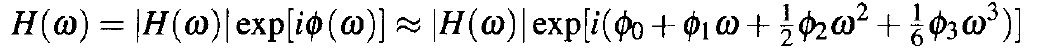where φm = dmφ/dωm (m = 0, 1, ...) is evaluated at the carrier frequency ω0. The constant phase φ0 and the time delay φ1 do not affect the pulse shape and can be ignored. The spectral phase introduced by the fiber can be compensated by choosing an optical filter such that φ2 = - β2L and φ3 = - β3L. The signal will be restored perfectly only if |H(ω)| = 1 and other higher-order terms in the above equation are negligible. The same optical filter can also reduce amplifier noise if its bandwidth is chosen suitably.

### Dispersion-Compensating Fibers

Optical filters whose transfer function has the form H(ω) = Hf*(L, ω) are not easy to design. The simplest solution is to use an especially designed fiber as an optical filter because it automatically has the desired form of the transfer function. This solution was suggested as early as 1980 and it provides an all-optical, fiber-based solution to the dispersion problem. During the 1990s, a special kind of fiber, known as the dispersion-compensating fiber (DCF), was developed for this purpose. Such fibers are routinely used for upgrading old fiber links or for installing new WDM fiber links. Such a scheme works well even when the nonlinear effects are not negligible as long as the average optical power launched into the fiber link is optimized properly.

#### 1. Conditions for Dispersion Compensation

Consider the situation shown in the figure above and assume that the optical bit stream propagates through two fiber segments of lengths L1 and L2, the second of which is the dispersion-compensating fiber (DCF). Each fiber has a transfer function of the form Hf(z, ω) expression above. After passing through the two fibers, the optical field is given by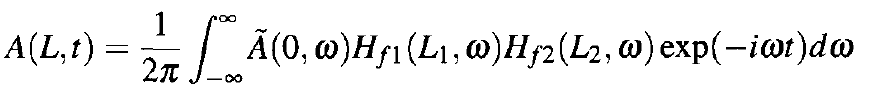where L = L1 + L2 is the total length. If the DCF is designed such that the product of the two transfer functions is 1, the pulse will fully recover its original shape at the end of DCF. If β2j and β3j are the GVD and third-order dispersion (TOD) parameters for the two fiber segments (j = 1, 2), the conditions for perfect dispersion compensation areThese conditions can be written in terms of the dispersion parameter D and the dispersion slope S (defined in this dispersion in single-mode fibers tutorial) as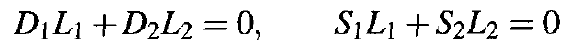The first condition is sufficient for compensating dispersion of a single channel since the TOD does not affect the bit stream much until pulse widths become shorter than 1 ps. Consider the upgrade problem for fiber links made with standard telecommunication fibers. Such fibers have D1 ≈ 16 ps/(km-nm) near the 1.55-μm wavelength within the C band. The equation above shows that a DCF must exhibit normal GVD (D2 < 0). For practical reasons, L2 should be as small as possible. This is possible only if the DCF has a large negative value of D2. As an example, if we use D1 = 16 ps/(km-nm) and assume L1 = 50 km, we need a 8-km-long DCF when D2 = -100 ps/(km-nm). This length can be reduced to 5 km if the DCF is designed to have D2 = -160 ps/(km-nm). In practice, DCFs with larger values of |D2| are preferred to minimize extra losses incurred inside a DCF that must also be compensated using an optical amplifier.

Consider now a WDM system. The second condition in the equation above must be satisfied if the same DCF must compensate dispersion over the entire bandwidth of a WDM system. The reason can be understood by noting that the dispersion parameters D1 and D2 in the equation above are wavelength-dependent. As a result, the single condition D1L1 + D2L2 = 0 is replaced with a set of conditions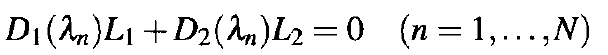where λn is the wavelength of the nth channel and N is the number of channels within the WDM signal. In the vicinity of the zero-dispersion wavelength of a fiber, D varies with the wavelength almost linearly. Writing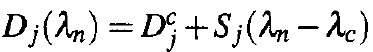in the equation above, where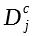is the value at the wavelength λc of the central channel, the dispersion slope of the DCF should satisfy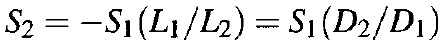This equation shows that the ratio S/D, called the relative dispersion slope, for the DCF should be equal to its value for the transmission fiber.

If we use typical values, D ≈ 16 ps/(km-nm) and S ≈ 0.05 ps/(km-nm2), we find that the ratio S/D is positive and about 0.003 nm-1 for standard fibers. Since D must be negative for a DCF, its dispersion slope S should be negative as well. Moreover, its magnitude should satisfy the equation above. For a DCF with D ≈ -100 ps/(km-nm), the dispersion slope should be about -0.3 ps/(km-nm2). The use of negative-slope DCFs offers the simplest solution to the problem of dispersion-slope compensation for WDM systems with a large number of channels. Indeed, such DCFs were developed and commercialized during the 1990s for dense WDM systems. In a 2001 experiment, broadband DCFs were used to transmit a 1-Tb/s WDM signal (101 channels, each operating at 10 Gb/s) over 9,000 km.

#### 2. Dispersion Maps

It is not necessary to employ just two fiber sections as shown in the first figure. In general, a fiber link may contain multiple types of fibers with different dispersion characteristics. The main impact of dispersion management from a mathematical standpoint is that the dispersion coefficients β2 and β3 become z-dependent as they change from one fiber section to the next. As long as the nonlinear effects remain negligible, we can still solve this equation. If we neglect the TOD effects for simplicity, the solution is modified to becomewhere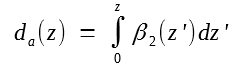represents the total accumulated dispersion up to a distance z.

Dispersion management requires that da(L) = 0 at the end of the fiber link so that A(L, t) = A(0, t). In practice, the accumulated dispersion of a fiber link is quantified throughIt is related to da as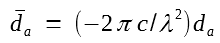The following figure shows three possible schemes for managing dispersion in long-haul fiber links. In each case, the accumulated dispersion is shown along the fiber link in a schematic fashion.In the first configuration, known as precompensation, the dispersion accumulated over the entire link is compensated at the transmitter end. In the second configuration, known as postcompensation, a DCF of appropriate length is placed at the receiver end. In the third configuration, known as periodic compensation, dispersion is compensated in a periodic fashion all along the link. Each of these configurations is referred to as a dispersion map, as it provides a visual map of dispersion variations along the link length. One can construct a variety of dispersion maps by combining several different fibers.

A natural question is which dispersion map is the best from a system's standpoint. For a truly linear system (no nonlinear effects), all three schemes are identical. In fact, any dispersion map for which da(L) = 0 at the end of a fiber link of length L would recover the original bit stream, no matter how much it became distorted along the way. However, nonlinear effects are always present, although their impact depends on the power launched into the fiber link. It turns out that the three configurations behave differently when nonlinear effects are included, and the system performance can be improved by adopting an optimized dispersion map.

#### 3. DCF Designs

There are two basic approaches to designing DCFs. In one case, the DCF supports a single mode and is fabricated with a relatively small value of the fiber parameter V. In the other approach, the V parameter is increased beyond the single-mode limit (V > 2.405) so that the DCF supports two or more modes. We consider both designs in this section.

In the single-mode design, the V parameter of the fiber is close to 1. This is accomplished by reducing the core size and modifying the refractive-index profile through doping of the core and the cladding regions. As discussed in the wave propagation in step-index single mode fibers tutorial, the fundamental mode of the fiber is weakly confined for V ≈ 1. As a large fraction of the mode propagates inside the cladding region, the waveguiding contribution to total dispersion is enhanced considerably, resulting in large negative values of D. A depressed-cladding design is often used in practice for making DCFs. Values of D below -100 ps/(km-nm) can be realized by narrowing the central core and adjusting the design parameters of the depressed cladding region surrounding the core. Dispersion slope S near 1550 nm can also be made negative and varied considerably by adjusting the design parameters to match the ratio S/D of the DCF to different types of transmission fibers.

Unfortunately, such DCFs suffer from two problems, both resulting from their relatively narrow core diameter.

First, they exhibit relatively high losses because a considerable fraction of the fundamental fiber mode reside in the cladding region (α ~ 0.5 dB/km). The ratio |D|/α is often used as a figure of merit for characterizing various DCFs. Clearly, this ratio should be as large as possible, and values >250 ps/(nm-dB) have been realized in practice.

Second, the effective mode area Aeff is only 20 μm2 or so for DCFs. As the nonlinear parameter γ ≡ 2πn2/(λAeff) is larger by a factor of 4 or so for DCFs compared with its value for standard fibers, the optical intensity is also larger at a given input power, and the nonlinear effects are enhanced considerably inside DCFs.

A practical solution for upgrading the exiting terrestrial lightwave systems operating over standard fibers consists of adding a DCF module (with 6-8 km of DCF) to optical amplifiers spaced apart by 60 to 80 km. The DCF compensates for GVD, while the amplifier takes care of fiber losses. This scheme is quite attractive but suffers from the loss and nonlinearity problems. Insertion losses of a DCF module often exceed 5 dB. These losses can be compensated by increasing the amplifier gain, but only at the expense of enhanced amplifier spontaneous emission (ASE). As input power should be kept relatively low to avoid the nonlinear effects, the transmission distance is limited by ASE noise.

Several new designs have been proposed to solve the problems associated with a standard DCF. In one design, shown schematically in figure (a) below, the DCF is designed with two concentric cores, separated by a ring-shaped cladding region.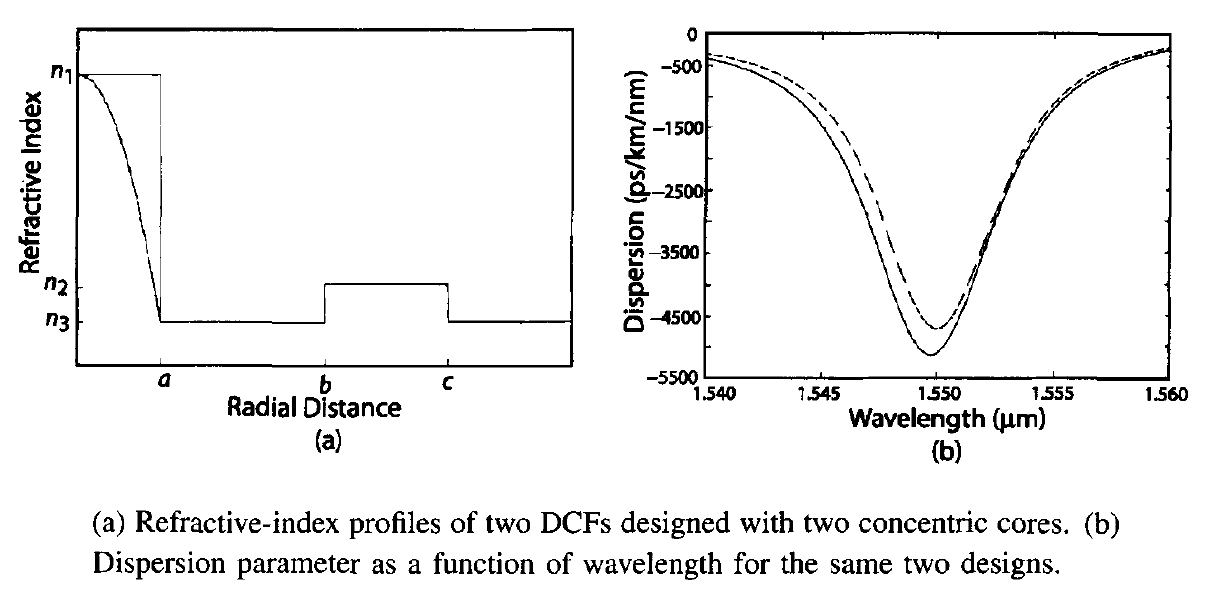The relative core-cladding index difference is larger for the inner core (Δi ≈ 2%) compared with the outer core (Δo ≈ 0.3%), but the core sizes are chosen such that each core supports a single mode. The three size parameters a, b, and c and the three refractive indices n1, n2, and n3 can be optimized to design DCFs with desired dispersion characteristics. The solid curve in figure (b) above shows the calculated values of D in the 1.55-μm region for a specific design with a = 1 μm, b = 15.2 μm, c = 22 μm, Δi = 2%, and Δo = 0.3%. The dashed curve corresponds to a parabolic index profile for the inner core. The mode diameter for both designs is about 9 μm, a value close to that of standard fibers. However, as shown in figure (b) above, the dispersion parameter can be as large as -5,000 ps/(km-nm) for such DCFs. It has proven difficult to realize such high values of D experimentally. Nevertheless, a DCF with D = -1,800 ps/(km-nm) was fabricated by 2000. For this value of D, a length of <1 km is enough to compensate dispersion accumulated over 100 km of standard fiber. Insertion losses are negligible for such small lengths.

The problems associated with a single-mode DCF can also be solved by using a fiber that support higher-order modes (HOMs). Such HOM fibers are designed with values of V > 2.5. They have almost the same loss as a single-mode fiber but can be designed such that the dispersion parameter D has large negative values for the second or higher-order modes. Indeed, values of D close to -770 ps/(km-nm) were measured as early as 1994 for elliptical-core DCFs. A 1-km length of such a DCF can compensate the GVD accumulated over 45 km, while adding relatively little to the total link loss or to the nonlinear degradation.

The use of few-mode DCFs requires a mode-conversion device capable of transferring optical signal from the fundamental mode to a higher-order mode supported by the DCF. Several such all-fiber devices have been developed. The all-fiber nature of the mode-conversion device is important to keep the insertion loss of the device to an acceptable level. Additional requirements on a mode converter are that it should be polarization-insensitive and should operate over a broad bandwidth. Almost all practical mode-conversion devices use an HOM fiber with a fiber grating that provides coupling between the two modes. The grating period Λ is chosen to match the effective-index difference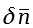between the two specific modes that are coupled by the grating (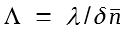) and is typically ~ 100 μm. Such gratings are called long-period fiber gratings.

The HOM fibers are inherently polarization sensitive, and their use requires polarization controllers in front of each dispersion-compensating module. This problem can be solved by using fibers oscillating in a few modes and employing the higher-order LP02 mode for dispersion compensation. Figure (a) below shows schematically such a DCF spliced in between two long-period fiber gratings that convert the LP01 mode into LP02 mode at the input end and then back into the LP01 mode at the output end. The mode converters are designed to be >99% efficient over the entire C band. The measured dispersion characteristics of such a 2-km-long DCF are shown in figure (b). The parameter D has a value of -420 ps/(km-nm) near 1,550 nm and this value changes considerably with wavelength because of a large dispersion slope associated with this fiber. This feature allows for broadband dispersion compensation by ensuring that the ratio S/D of the DCF is close to the fiber used for transmitting data. Other useful features of such a DCF are that the device is polarization-insensitive, exhibits relatively low insertion loss (<3.7 dB), and offers dispersion compensation over the entire C band.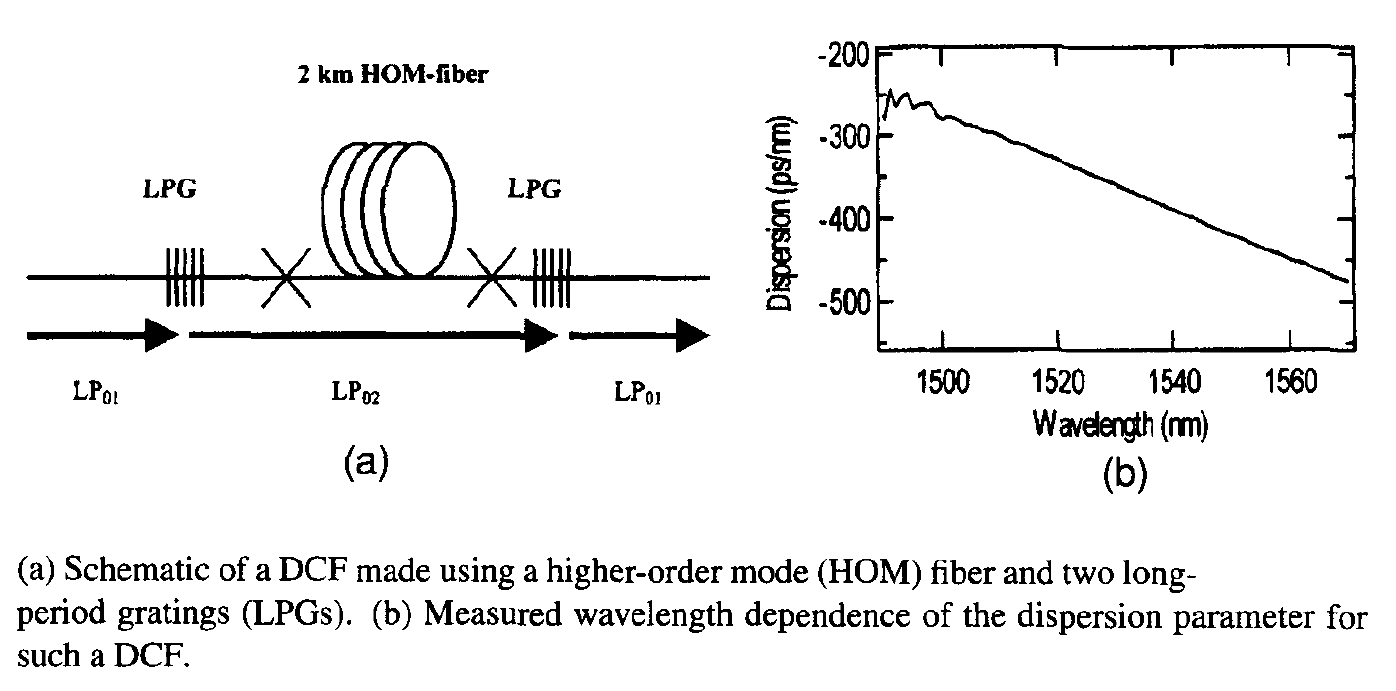Another approach to DCF design makes use of photonic crystal fibers. Such fibers contain a two-dimensional array of air holes around a central core that modifies their dispersion characteristics substantially, depending on the size of air holes and the spacing among them. Dual-core photonic-crystal fibers can provide large negative values of D with dispersion slopes that make them quite suitable for broadband dispersion compensation in WDM systems. Figure (a) below shows the cross section of one design for such fibers. It contains an inner ring of air holes with a smaller diameter d1 that is surrounded by multiple rings of air holes with a larger diameter d2. Calculated values of the dispersion parameter D as a function of wavelength are shown in figure (b) below for three values of d2 when d1 = 1.68 μm. Dispersion compensation over a much larger bandwidth can be realized with further modifications in the design of such fibers.